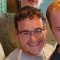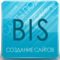# More ExamplesLast updated Dec 21st, 2019 | Page history | Improve this page | Report an issue

## Goal¶

This page contains examples that juxtapose MySQL database tables with their xPDO XML schema counterparts in order to teach developers how to define the foreign-key relationships between tables in xPDO XML schemas by using a series of common database relational patterns as examples.

Database relations can be complex, so it's no surprise that the XML schema files that describe those relations reflect that complexity. Although xPDO schema files already exist for built-in MODX tables inside of core/model/schema/modx.mysql.schema.xml, we don't recommend that developers rely on those XML files as their only examples of how to relate tables because they are often too complex to be used for educational purposes.

Remember that xPDO abstracts the database, so it's entirely possible that your model is something other than a traditional database, but for the sake of familiarity and clarity, the examples here assume you are using a MySQL database for your model. In general, it's recommended that you design your snippets/plugins etc. using a traditional database before abstracting it using xPDO.

FYI Once you've created a valid XML schema file, xPDO can generate PHP class files and database tables; it is bi-directional. The purpose of this page is to juxtapose the xPDO XML schema to MySQL database tables. Some developers may prefer to write the XML schema file by hand and then let xPDO generate the tables and the class files. Other developers may prefer to first create the database tables, then reverse engineer the XML schema and the class files.

## One to One¶

A one-to-one relationship exists when two tables use the same primary key. Architecturally, this means that the data could theoretically live in a single table, but for whatever reason, the data is separated into two (or more) tables.

The tricky thing about one-to-one relationships is that both tables are not equal. Like the movie Highlander, there can be only one primary table: you must decide which table is the primary (or master) table, and which is the secondary (or slave) table.

A good example of this type of relationship exists in the MODX database between the users and the user_attributes tables: the users table is the primary table, and the user_attributes is the secondary table. If you delete a user from the users table, the extra attributes in the user_attributes table should also be deleted, but the opposite is not necessarily true. The documentation on relationships stresses this primary/secondary relationship.

When defining relationships, you must first learn about aggregate and composite relationships; you may not understand the usage of these particular words, but when defining this type of relationship, simply remember the following:

• The primary table's XML definition lists a composite relationship to the the secondary table.
• The secondary table's XML definition lists an aggregate relationship to the primary table.

For this example, we are mimicking the MODX tables where we have one table for users and a secondary table that stores additional information about those users, named userdata.

FYI Unlike some ORMs (e.g. Doctrine) and even unlike MySQL's foreign-key definitions, xPDO defines a relationship on both sides. You tell the children who their parents are and you also tell the parents who their children are. E.g. the parent object contains a composite relationship and the child object contains an aggregate relationship. This ensures that everybody knows who they're related to.

MySQL Table Definitions

Here are abbreviated MySQL table definitions:

``````CREATE TABLE `users` (
`user_id` int(11) NOT NULL AUTO_INCREMENT,
`bio` text,
`joindate` date DEFAULT NULL,
PRIMARY KEY (`user_id`)
) ENGINE=MyISAM;
CREATE TABLE `userdata` (
`userdata_id` int(11) NOT NULL AUTO_INCREMENT,
`age` tinyint(3) unsigned DEFAULT NULL,
PRIMARY KEY (`userdata_id`)
) ENGINE=MyISAM;
``````

This MySQL query will show all data for users (including info from the primary user table, and also from the secondary userdata table):

``````SELECT users.*, userdata.*
``````

XML Schema

And here's the corresponding XML definitions:

``````<object class="Users" table="users" extends="xPDOObject">
<field key="user_id" dbtype="int" precision="11" phptype="integer" null="false" index="pk"  generated="native" />
<field key="username" dbtype="varchar" precision="255" phptype="string" null="true" />
<field key="bio" dbtype="text" phptype="string" null="true" />
<field key="joindate" dbtype="date" phptype="date" null="true" />
<index alias="PRIMARY" name="PRIMARY" primary="true" unique="true">
<column key="user_id" collation="A" null="false" />
</index>
<composite alias="Userdata" class="Userdata" local="user_id" foreign="userdata_id" cardinality="one" owner="local" />
</object>
<object class="Userdata" table="userdata" extends="xPDOObject">
<field key="userdata_id" dbtype="int" precision="11" phptype="integer" null="false" index="pk"  generated="native" />
<field key="age" dbtype="tinyint" precision="3" attributes="unsigned" phptype="integer" null="true" />
<index alias="PRIMARY" name="PRIMARY" primary="true" unique="true">
<column key="userdata_id" collation="A" null="false" />
</index>
<aggregate alias="Users" class="Users" local="userdata_id" foreign="user_id" cardinality="one" owner="foreign" />
</object>
``````

Sample Snippet Code

If you were to access this data in a Snippet, you might do something like the following. This assumes that your package name is one_to_one

``````<?php
\$base_path = MODX_CORE_PATH . 'components/one_to_one/';
\$user = \$modx->getObject('Users', array('user_id' => 1 ) );
\$userdata = \$user->getOne('Userdata');
\$output = '';
\$output .= \$userdata->get('age');
return \$output;
?>
``````

## One to Many¶

This is a common pattern that occurs when a secondary table contains a foreign key. For example, you might have a primary table containing blog posts, and a secondary table containing comments. Each blog post might have zero or many comments, but each comment can belong to one and only one blog post.

This is the same type of relationship that exists in MODX between pages and templates: a single template might be used by hundreds of pages, but a page can only use a single template.

MySQL Table Definitions

``````CREATE TABLE `blogposts` (
`blogpost_id` int(11) NOT NULL AUTO_INCREMENT,
`content` text,
PRIMARY KEY (`blogpost_id`)
) ENGINE=MyISAM;
`comment_id` int(11) NOT NULL AUTO_INCREMENT,
`blogpost` int(11) DEFAULT NULL,
`comment` text,
PRIMARY KEY (`comment_id`)
) ENGINE=MyISAM;
``````

XML Schema

``````<object class="Blogposts" table="blogposts" extends="xPDOObject">
<field key="blogpost_id" dbtype="int" precision="11" phptype="integer" null="false" index="pk"  generated="native" />
<field key="content" dbtype="text" phptype="string" null="true" />
<index alias="PRIMARY" name="PRIMARY" primary="true" unique="true">
<column key="blogpost_id" collation="A" null="false" />
</index>
</object>
<field key="comment_id" dbtype="int" precision="11" phptype="integer" null="false" index="pk"  generated="native" />
<field key="blogpost" dbtype="int" precision="11" phptype="integer" null="true" />
<field key="comment" dbtype="text" phptype="string" null="true" />
<index alias="PRIMARY" name="PRIMARY" primary="true" unique="true">
<column key="comment_id" collation="A" null="false" />
</index>
<aggregate alias="Blogposts" class="Blogposts" local="blogpost" foreign="blogpost_id" cardinality="one" owner="foreign" />
</object>
``````

Sample Snippet Code

Here is some sample Snippet code. It assumes your package name is one_to_many:

``````<?php
\$base_path = MODX_CORE_PATH . 'components/one_to_many/';
\$output = '';
\$blogpost = \$modx->getObject('Blogposts', array('blogpost_id' => 1 ) );
\$output .= \$blogpost->get('content');
foreach ( \$comments as \$c )
{
\$output .= \$c->get('comment');
}
return \$output;
``````

## Many to Many: Joining Tables¶

Another common database pattern involves the use of join tables. This type of relationship is seen frequently when using taxonomies such as "categories" or "tags": e.g. a single post can be "tagged" with multiple terms, and each tag can likewise be associated with multiple posts.

This type of relationship infers three database tables: blogposts, tags, and the joining table blogposts_tags. The trick here is that two of the tables are acting as primary tables: both the blogposts and the tags table will contain composite definitions that point to the blogposts_tags.

MySQL Table Definitions

``````CREATE TABLE `blogposts` (
`blogpost_id` int(11) NOT NULL AUTO_INCREMENT,
`content` text,
PRIMARY KEY (`blogpost_id`)
) ENGINE=MyISAM;
CREATE TABLE `tags` (
`tag_id` int(11) NOT NULL AUTO_INCREMENT,
`name` varchar(255) DEFAULT NULL,
PRIMARY KEY (`tag_id`)
) ENGINE=MyISAM;
/* The Join Table: */
CREATE TABLE `blogposts_tags` (
`id` int(11) NOT NULL AUTO_INCREMENT,
`blogpost` int(11) DEFAULT NULL,
`tag` int(11) DEFAULT NULL,
PRIMARY KEY (`id`)
) ENGINE=MyISAM;
``````

XML Schema

Note the the following schema still contains the composite relationship for the Comments table.

``````<object class="Blogposts" table="blogposts" extends="xPDOObject">
<field key="blogpost_id" dbtype="int" precision="11" phptype="integer" null="false" index="pk"  generated="native" />
<field key="content" dbtype="text" phptype="string" null="true" />
<index alias="PRIMARY" name="PRIMARY" primary="true" unique="true">
<column key="blogpost_id" collation="A" null="false" />
</index>
<composite alias="BlogpostsTags" class="BlogpostsTags" local="blogpost_id" foreign="blogpost_id" cardinality="many" owner="local" />
</object>
<object class="Tags" table="tags" extends="xPDOObject">
<field key="tag_id" dbtype="int" precision="11" phptype="integer" null="false" index="pk"  generated="native" />
<field key="name" dbtype="varchar" precision="255" phptype="string" null="true" />
<index alias="PRIMARY" name="PRIMARY" primary="true" unique="true">
<column key="tag_id" collation="A" null="false" />
</index>
<composite alias="BlogpostsTags" class="BlogpostsTags" local="tag_id" foreign="tag_id" cardinality="many" owner="local" />
</object>
<object class="BlogpostsTags" table="blogposts_tags" extends="xPDOSimpleObject">
<field key="blogpost" dbtype="int" precision="11" phptype="integer" null="true" />
<field key="tag" dbtype="int" precision="11" phptype="integer" null="true" />
<index alias="PRIMARY" name="PRIMARY" primary="true" unique="true">
<column key="id" collation="A" null="false" />
</index>
<aggregate alias="Tags" class="Tags" local="tag" foreign="tag_id" cardinality="one" owner="foreign" />
<aggregate alias="Blogposts" class="Blogposts" local="blogpost" foreign="blogpost_id" cardinality="one" owner="foreign" />
</object>
``````

Sample Snippet Code

The following example assumes that the package is named many_to_many. Note that the logic displayed here traces the relationships precisely. In this example, we load up a blogpost, then trace it through the joining table to its tags. Arguably, this isn't any easier than writing a JOIN statement in MySQL.

``````<?php
\$base_path = MODX_CORE_PATH . 'components/many_to_many/';
\$output = '';
\$blogpost = \$modx->getObject('Blogposts', array('blogpost_id' => 1 ) );
\$blopost_tags = \$blogpost->getMany('BlogpostsTags');
foreach ( \$blopost_tags as \$bt )
{
\$tag = \$bt->getOne('Tags');
\$output .= \$tag->get('name');
}
return \$output;
``````

## Parent ID: Self Join¶

Another common pattern used to indicate hierarchy is the self-join. This is when one column in a table contains a reference to that table's own primary key. We are familiar with this in the MODX database when we put pages into folders: there is a parent/child relationship where each page may be the child of another page.

In this example, we are going to demonstrate how a table can define hierarchical categories using a parent/child relationship. If a parent_id is defined for a row in our categories table, it means that the row represents a sub-category of the parent.

MySQL Table Definitions

``````CREATE TABLE `categories` (
`category_id` int(11) NOT NULL AUTO_INCREMENT,
`parent_id` int(11) DEFAULT NULL,
`name` varchar(255) DEFAULT NULL,
`seq` smallint(4) DEFAULT NULL,
PRIMARY KEY (`category_id`)
) ENGINE=MyISAM;
``````

XML Schema

In order to define this relationship in xPDO XML, we must add 2 aggregate relationships to the object:

``````<object class="Categories" table="categories" extends="xPDOObject">
<field key="category_id" dbtype="int" precision="11" phptype="integer" null="false" index="pk"  generated="native" />
<field key="parent_id" dbtype="int" precision="11" phptype="integer" null="true" />
<field key="name" dbtype="varchar" precision="255" phptype="string" null="true" />
<field key="seq" dbtype="smallint" precision="4" phptype="integer" null="true" />
<index alias="PRIMARY" name="PRIMARY" primary="true" unique="true">
<column key="category_id" collation="A" null="false" />
</index>
<aggregate alias="Parent" class="Categories" local="parent_id" foreign="category_id" cardinality="one" owner="foreign" />
<composite alias="Children" class="Categories" local="category_id" foreign="parent_id" cardinality="many" owner="local" />
</object>
``````

Sample Snippet Code

In this example, our package is named parent_child_example. Notice that the getMany method relies on the alias defined for that relationship.

``````<?php
\$base_path = MODX_CORE_PATH . 'components/parent_child_example/';
\$output = '';
\$category = \$modx->getObject('Categories', array('category_id' => 1 ) );
\$subcategories = \$category->getMany('Children');
\$output .= \$category->get('content');
foreach ( \$subcategories as \$sc )
{
\$output .= \$sc->get('name');
}
return \$output;
``````

## Using Field Aliases (xPDO 2.2+ only)¶

In this example, we are setting an alias of postalcode for the zip field from the storefinder model.

XML Schema

The field alias definition is simply defined using the `alias` element.

``````<object class="sfStore" table="sfinder_stores" extends="xPDOSimpleObject">
<field key="name" dbtype="varchar" precision="100" phptype="string" null="false" default="" index="index" />
<field key="address" dbtype="varchar" precision="255" phptype="string" null="false" default="" />
<field key="city" dbtype="varchar" precision="255" phptype="string" null="false" default="" />
<field key="state" dbtype="varchar" precision="255" phptype="string" null="false" default="" />
<field key="zip" dbtype="varchar" precision="10" phptype="string" null="false" default="0" index="index" />
<field key="country" dbtype="varchar" precision="20" phptype="string" null="false" default="" />
<field key="phone" dbtype="varchar" precision="20" phptype="string" null="false" default="" />
<field key="fax" dbtype="varchar" precision="20" phptype="string" null="false" default="" />
<field key="active" dbtype="int" precision="1" attributes="unsigned" phptype="integer" null="false" default="0" />
<alias key="postalcode" field="zip" />
<index alias="name" name="name" primary="false" unique="false" type="BTREE">
<column key="name" length="" collation="A" null="false" />
</index>
<index alias="zip" name="zip" primary="false" unique="false" type="BTREE">
<column key="zip" length="" collation="A" null="false" />
</index>
</object>
``````

Sample Snippet Code

The alias postalcode is now accessible as a field of an sfStore object in xPDO. It is simply a reference to the value of the zip field.

``````<?php